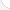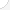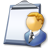Veterinary technologists and techniciansDescription: Veterinary Technologists and Technicians perform many of the same duties for a veterinarian that a nurse would for a physician, including laboratory and clinical work such as urine and blood tests. They may work in private vets' offices, zoos, research facilities, and other places where veterinarians work.Source: Bureau of Labor Statistics, U.S. Department of Labor, Occupational Outlook Handbook, 2008-09 Edition at http://www.bls.gov/OCO/Complete Job Profile: http://www.bls.gov/oco/ocos183.htmSalary: \$15,001 to \$23,000 per yearComments:I, too, was one of the naysayers on math....hated it! But I use it every day! My dad insisted on us being able to do simple math in our heads without *gasp!!* calculators! I still amaze my co-workers when I do simple calculations in my head and give them the answer while they are still writing out the equation. Of course, when doing dose calculations for medicines, it is always wise to do that 'check your answer' thing! LOL! lynnzoi@yahoo.com Lynn D

There are 36 math topics Veterinary technologists and technicians need to know.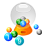Basic Math / Algebra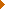FractionsDecimalsRatio and ProportionPercentCustomary MeasurementMetric MeasurementMeasurement ConversionBasic ProbabilityBasic StatisticsStatistical GraphingPowers and RootsOther Number BasesNegative NumbersScientific NotationBasic Problem Solving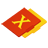First-Year AlgebraUsing FormulasLinear EquationsOperations with PolynomialsAlgebraic Representation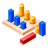GeometryBasic TerminologyAngle MeasurementParallel LinesGeometric MeanPythagorean TheoremCirclesAreaVolumeMake/Use 3D Drawings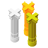Second-Year Algebra / TrigonometryVariation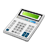Other TopicsBasic Calculator UseScientific Calculator UseComputer UseGroup Problem SolvingMental MathInductive/Deductive ReasoningMath Communications